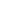Get Car Insurance

Did you know you can insure your car for as low as 2% of your car value?Interest Rate, Definitin & types

What is interest?

Interest is the amount you are asked to pay to borrow money in addition to paying back the original amount you borrowed. I.e. If you borrow LE 100 and are asked to pay back LE 110, then LE 100 is the principal and LE 10 is the interest.

What is interest rate & how do you calculate it?

Interest rate is an expression of how much interest you will be charged for a specific period of time and is expressed in percentage (i.e. 14.00%), usually the typical time period used is 1 year but that is not always the case.

Using the example above, assuming you borrowed the LE 100 for 1 year and were asked to pay back LE 110 then the interest rate of the LE 10 interest is 10.00% per year.

While most products quote interest rates annually, there is one notable exception which is Credit Cards that typically quote interest rates on a monthly basis

What’s the difference between decreasing and flat interest rates?

First to dispel a myth, a flat interest rate is not cheaper or different than a decreasing interest rate, they are both actually different ways to present the same thing. Let’s explain it below:

·         Decreasing Interest Rate: A decreasing interest rate is the percentage that will be charged against the outstanding amount only. I.e. if I am borrowing at a decreasing interest rate of 12.00% and I currently owe LE 100.0, then I will be charged LE 12.0 for the year. For the second year, assume I paid LE 20.0 from the principal and only owe LE 80.0, I will then be charged LE 9.6 for that year.

·         Flat Interest Rate: A flat interest rate is expressed as the average that you will pay across the period of the loan. So taking the previous example, let’s assume the loan is LE 100,0 paid over 5 years equally, I will be charged as follows:

o   LE 12.0 interest amount Year 1

o   LE 9.6 interest amount Year 2

o   LE 7.2 interest amount Year 3

o   LE 4.8 interest amount Year 4

o   LE 2.4 interest amount Year 5

o   Total of LE 36,0 interest amount for the period of 5 years.

The flat interest rate in the example we used would be calculated as (Total interest amount divided by principal amount divided by a number of years). So If we take LE 36 divided by our principal of LE 100 divided by 5 years which would give us a flat rate of 7.20%. So basically, in this example, a decreasing interest rate of 12.00% is equal to a flat rate of 7.20%.

The most important thing to remember is to understand what type of rate the bank is quoting you and to make sure you always compare the same type of interest rate, you can always double-check by checking the amount of the instalment.

All the interest rates shown for loan products on Faydety are decreasing interest rates, if you want to know the flat rate just click on “More Details” below the loan you are looking at.

What is compounding interest?

Compounding interest is an important concept to understand when looking at deposits. As explained earlier the interest rate is typically expressed annually, so to flip the example around if I deposit LE 100.0 for one year at an interest rate of 9.00% I will get back LE 109.0 as a total payment, LE 100.0 my principal and LE 9.0 in interest.

In deposits, I have the option of getting paid my interest every 3 months, every 6 months, every year or at the end of the period. Compounding interest relates to if I decide to deposit money for more than a year and want to be paid my interest amount at the end of the period. So back to our example; if I deposit LE 100.0 at the same interest rate as above for three years, at the end of the first year I have earned LE 9.0 in interest but I have not taken that money. So when I come to calculate my principal for the second year, it is LE 109.0, not LE 100.0. As such the interest I earn is on the new amount which is the principal and the interest earned last year, in this case, LE 9.8.

The Money Blog

Our blog makes understanding finance a breeze…

Currency Rates in Egypt at The 23rd of January 2022

Banking Products

Personal Loans

Car Loans

Micro Finance

Credit Cards

Saving Deposits